# NCERT Solutions for Class 12 MathsNCERT Solutions for Class 12 Maths is written to assist students that are making preparations to sit for various board exams including JEE Mains, VITEEE, BITSAT, etc. This offers sound knowledge plus the understanding of every significant concept that is highlighted in every chapter in the NCERT textbook for Class 12 Maths. We have 13 chapters from the NCERT Maths textbook. All the problems plus their comprehensive solutions are provided. Additionally, the solutions to the questions have been devised by the best teachers to assist students to get basic concepts faster and better.

For one to get entry into the best colleges, students need to perform best. This may push them to be under pressure & be stressed & demotivated. Moreover, students don’t need to fear anymore because, with the assistance of NCERT books, they will easily attain their target scores.

NCERT Textbooks for Class 12 for Maths have every bit plus a piece of the chapters to ensure that nothing is left for the students to practice. Maths entails solutions and every question solved contains a step-by-step process stated to reach an answer. This offers students an idea of formulas plus equations that are to be utilized for other types of questions.

## NCERT Solutions for Class 12 Maths – Chapter-Wise

### Chapter 1: Relations & Functions

Chapter one of NCERT Solutions for Maths for Class 12 is about relations & functions. Here you will learn about kinds of relations, varieties of functions, invertible function & functions composition, binary operations plus miscellaneous illustrations. You will also get exercises solution linking to the topics that are covered in this chapter.

Topics that are covered in Maths for Class 12 Chapter One (Relations & Functions) for First Term include:

Kinds of relations: symmetric, reflexive, transitive & equivalence relations. One-to-one plus onto functions.

#### Topics and sub-topics of the Relations & Function chapter

• 1.1 Introduction
• 1.2 Types of Relations
• 1.3 Types of Functions
• 1.4 Composition of Functions and Invertible Function
• 1.5 Binary Operations

#### FAQ

What are relations and functions in class 12?

It is a special kind of relation(a set of ordered pairs) which obeys a rule, i.e. every y-value should be connected to only one y-value. Mathematically, “a relation f from a set A to a set B is said to be a function if every element of set A has one and only one image in set B”.

How many exercises are there in relation to and function class 12?

NCERT Solutions for Class 12 Maths Chapter 1 Relations and Functions has 55 questions in 4 exercises.

What are into functions of Class 12?

Onto (Surjective) Function. A function f: X -> Y is said to be an onto function, if every element of Y is an image of some element of set X under f, i.e. for every y ∈ Y there exists an element x in X such that f(x) = y. Properties: The range of functions should be equal to the codomain.

What is function class 12 computer science?

A function can be defined as a single related task, which is written as a block of codes. It only runs when it is called. We can pass data to the function when we call a function, these are known as parameters. A function can return data as a result of using the return statement.

## Chapter 2: Inverse Trigonometric Functions

In chapter two, Inverse Trigonometric Functions will be covered. This offers an account of different topics like the remarks centered on inverse trigonometric functions basic concepts, inverse trigonometric functions properties & miscellaneous illustrations. All the concepts are well explained via examples. Solutions to exercises from this chapter will be accessed.

Topics Covered in the First Term for Class 12 Maths Chapter Two (Inverse Trigonometric Functions) include definition, domain, range, and principal value branch.

## Chapter 3: Matrix

Here you will learn about the properties of important Mathematics tools, namely Matrices. Here students will be able to study and understand the basic concept of Matrices & Matrix Algebra. the students will know matrix definition, matrices types, matrices equality, matrices operations including addition & multiplication of a matrix by a scalar, scalar multiplication properties, matrix addition properties, matrices multiplication, and matrices multiplication properties. Moreover, the student will also learn how to transpose a matrix, matrix transpose properties, symmetric & skew-symmetric matrices, matrix transformation or elementary operation, matrix inverse by elementary operations & miscellaneous illustrations.

Subtopics covered include; concept, order, notation, equality, kinds of matrices, zero & identity matrix, matrix transpose, symmetric, skew-symmetric matrices, etc.

## Chapter 4: Determinants

This chapter is a continuation of the topic of Matrix. The chapter deals with a significant part of Matrices that’s namely Determinants. Here you will find determinants of orders 3×3 plus their cofactors & inverses.

The chapter also speaks about determinants applications, including calculating an area of triangles, constancy of linear equations sets, and adjoint of matrices.

Topics and sub-topics for Chapter 4 Determinants Class 12

## Chapter 5: Continuity & Differentiability

This chapter picks up after the Functions Differentiation that’s introduced in Class 11. This chapter explores significant ideas about Continuity, and Differentiability & creates a relationship between them. Furthermore, it also deals with the continuity & differentiability of Functions of Inverse Trigonometric. Here, you will also be introduced to exponential & logarithmic functions.

Topics and sub-topics included in the Continuity and Differentiability Chapter Class 12

• Introduction
• Algebra of continuous functions
• Differentiability
• Derivatives of composite functions
• Derivatives of implicit functions
• Derivatives of inverse trigonometric functions
• Exponential and Logarithmic Functions
• Logarithmic Differentiation
• Derivatives of Functions in Parametric Forms
• Second-Order Derivative
• Mean Value Theorem
• Summary

## Chapter 6: Applications of Derivatives

Here the student will get details about derivatives definition, rate-of-change of quantities, decreasing & increasing functions, normal & tangents, maxima & minima, approximations, test for the first derivative, maximum & minimum values about a function in closed interval & miscellaneous illustrations.

Topics and sub-topics included in the Applications of Derivatives Chapter 6 Class 12

## Chapter 7: Integrals

Differential Calculus is dependent on a derivative idea. Derivatives came for the issue of defining tangent lines to graphs of functions & calculating the slope of such lines. Furthermore, Integral Calculus simplifies the issue of defining & calculating an area that’s bounded by a function. Subtopics available in the integrals chapter include indefinite integrals introduction, integration (inverse of differentiation), geometrical interpretation, & properties. Other subtopics include integration & differentiation comparison, and integration methods (substitution, by parts, partial fraction, & trigonometric identities).

Topics and sub-topics included in the Integral Chapter 7 Class 12

## Chapter 8: Integrals Applications

This chapter is a continuation of integrals. It starts with a definition, an area on simple curves, an area in between 2 curves, an area on a region surrounded by a curve & a line, & miscellaneous illustrations.

Topics and sub-topics included in the Integrals Applications Chapter 8 Class 12

## Chapter 9: Differential Equations

In this chapter, the student will study some concepts that are related to differential equations, differential equations formation, general & particular solutions of a differential equation, the number of methods that solve questions centered on first-order- first-degree differential equations & differential equations applications in various areas. In this chapter, the student will have a total of six exercises to practice on. Implementation of differential equations in a plethora of applications in every other subject & area.

Topics and sub-topics included in the Differential Equations Chapter 9 Class 12

## Chapter 10: Vector Equations

In this chapter the student will encounter vector quantities, that’s the quantities all both magnitude & direction. Vector algebra summarizes addition rules of vector quantities, plus other significance properties. The student will also learn about the varieties of vectors including zero, unit, collinear & equal vectors.

Other different topics which are to be discussed in this chapter include negative vectors, vectors components & how one can multiply a vector with a scalar. Moreover, the student will also study section formulae, the dot-product of 2 vectors, vector joining 2 points, a product of 2 vectors, vector projection on a line, and the cross-product of two vectors.

Topics and sub-topics included in the Vector Equations Chapter 10 Class 12

## Chapter 11: Three-Dimensional Geometry

In this chapter, the student will study the direction ratios & direction cosines of a line that joins two points & also the equations of a line & plane in space on various conditions. Also, the student will get to understand the angle between two lines, a line & a plane, 2 planes, the smallest distance between 2 skew lines & the distance from a plane to a point.

Furthermore, the student will also get to know about cartesian equations & vector line equations, skew & coplanar lines.

Topics and sub-topics included in the Three-Dimensional Geometry Chapter 11 Class 12

## Chapter 12: Linear Programming

In this chapter, the students will be led through linear programming. Some of the concepts that are covered in this chapter include linear programming-problem & its mathematical formulation, graphical way of solving linear programming, mathematical formulation, and different varieties of linear programming with miscellaneous illustrations. The subtopics include an introduction, terminologies like constraints, optimization, objective function, and different kinds of linear programming problems. Graphical way solutions in two variables, infeasible & feasible regions (bounded), infeasible & feasible solutions, best feasible solutions.

Topics and sub-topics included in the Linear Program Chapter 11 Class 12

## Chapter 13: Probability

This chapter will take you to significant concepts of conditional probability about an event provided that a different event occurred, in turn, which will clarify Bayes’ theorem, probability multiplication rule & independence of events.

Furthermore, a student will get about five exercises, where you’ll learn a significant concept about random variables & their probability distribution, variance, and the mean of a probability distribution. Here, the student will also learn about the partition of a sample space, Bernoulli trials, total probability theorem, & binomial distribution.

Topics and sub-topics included in the Probability Chapter 13 Class 12

## Significant Features of CBSE Notes NCERT Solutions for Maths for Class 12

• Students easily practice & revise concepts by referring to the solutions.
• Saves time by providing every important concept & formula in a suitable sequence.
• NCERT Maths Solutions consists of significant tips, important for answering normal questions accurately.
• The solutions are very helpful for fast revisions as you prepare for your exams.

## How to improve NCERT Solutions for Class 12 Maths mark?

1. Understand Mathematics the concepts
2. Practice regularly
3. Solve previous year’s question papers
5. Use diagrams and graphs
6. Time management
7. Stay focused

## CBSE Sample Papers for Class 12 Maths With Solutions with set

Check below all math set solutions to improve your skill and marks in your upcoming exam.

### CBSE Sample Papers for Class 12 Maths Set 1

Time Allowed: 3 Hours
Maximum Marks: 80

Instructions:

• This Question paper contains five sections A, B, C, D, and E. Each section is compulsory. However, there are internal choices in some questions.
• Section A has 18 MCQs and 2 Assertion-Reason-based questions of 1 mark each.
• Section B has 5 Very Short Answer (VSA) type questions of 2 marks each.
• Section C has 6 Short Answer (SA) type questions of 3 marks each.
• Section D has 4 Long Answer (LA) type questions of 5 marks each.
• Section E has 3 sources based/case based/passage based/integrated units of assessment (4 marks each) with sub-parts.

## Section – A (20 Marks)

(Multiple Choice Questions Each question carries 1 mark)

#### Question 1.

If A = [aij] is a skew-symmetric matrix of order n, then 

(a) aij = 1aji ∀ i, j
(b) aij ≠ 0 ∀ i, j
(c) aij = 0, where i = j
(d) aij ≠ 0 where i = j

Solution: (c) aij = 0, where i = j

Explanation:

In a skew-symmetric matrix, the (i, j)th element is negative of the (j, i)th element. Hence, the (i, j)th element = 0
By definition of the skew-symmetric matrix, diagonal elements of a skew-symmetric matrix are all 0.

#### Question 2.

If A is a square matrix of order 3, |A’| = -3, then |AA’| = 

(a) 9
(b) -9
(c) 3
(d) -3

Solution: (a) 9

Explanation: |AA’| = |A||A’| = (-3) (-3) = 9

#### Question 3.

The area of a triangle with vertices A, B, and C is given by 

(a) |AB−→−×AC−→−|
(b) 12|AB−→−×AC−→−|
(c) 14|AC−→−×AB−→−|
(d) 18|AC−→−×AB−→−|
Solution:
(b) 12|AB−→−×AC−→−|

Explanation: The area of the parallelogram with adjacent sides AB and AC = |AB−→−×AC−→−|.
Hence, the area of the triangle with vertices A, B, C = 12|AB−→−×AC−→−|

#### Question 4.

The value of ‘k’ for which the function f(x) = {1−cos4x8x2k, if x≠0 if x=0 is continuous at x = 0 is 
(a) 0
(b) -1
(c) 1
(d) 2

Solution: (c) 1

Explanation: The function f is continuous at x = 0 if limx→0f(x)=f(0)

Question 5.

If f'(x) = x + 1x, then f(x) is 

(a) x2 + log|x| + C
(b) x22 + log |x| + C
(c) x2 + log |x| + C
(d) x2 – log |x| + C

Solution: (b) x22 + log |x| + C

Explanation: x22 + log |x| + C (∵ f(x) = ∫(x+1x)dx)
Given, f'(x) = x + 1x
By integrating both sides, we get
f'(x) = x22 + log x + C

#### Question 6.

If m and n, respectively, are the order and the degree of the differential equation ddx[(dydx)]4 = 0, then m + n = 

(a) 1
(b) 2
(c) 3
(d) 4

Solution:

(c) 3

Explanation:

The given differential equation is 4(dydx)3d2ydx2=0
Here, m = 2 and n = 1
Hence, m + n = 3

Question 7.

The solution set of the inequality 3x + 5y < 4 is 

(a) an open half-plane not containing the origin
(b) an open half-plane containing the origin
(c) the whole XY-plane not containing the Line 3x + 5y = 4
(d) a closed half-plane containing the origin

Solution:

(b) an open half-plane containing the origin.

Explanation:

The strict inequality represents an open half plane and it contains the origin as (0, 0) satisfies it

Question 8.

The scalar projection of the vector 3i^−j^−2k^ on the vector i^+2j^−3k^ is 

(a) 714√
(b) 714
(c) 613
(d) 72

Solution: (a) 714√

Explanation:

Scalar Projection of 3i^−j^−2k^ on vector i^+2j^−3k^ = (3i^−j^−2k^)⋅(i^+2j^−3k^)|i^+2j^−3k^|=714√

Question 9.

The value of ∫32xx2+1dx is 

(a) log 4
(b) log32
(c) 12log 2
(d) log94

Solution:

(c) 12log 2

Explanation: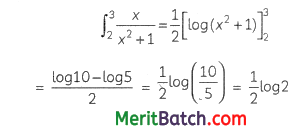Question 10.

If A, and B are non-singular square matrices of the same order, then (AB-1)-1 = 

(a) A-1B
(b) A-1B-1
(c) BA-1
(d) AB

Solution:

(c) BA-1

Explanation:

(AB-1)-1 = (B-1)-1 A-1 = BA-1

Question 11.

The corner points of the shaded unbounded feasible region of an LPP are (0, 4), (0.6, 1.6) and (3, 0) as shown in the figure. The minimum value of the objective function Z = 4x + 6y occurs at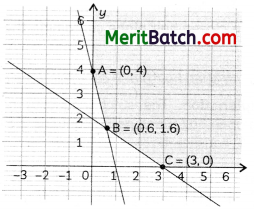(a) (0.6, 1.6) only
(b) (3, 0) only
(c) (0.6, 1.6) and (3, 0) only
(d) at every point of the line segment joining the points (0.6, 1.6) and (3, 0)

Solution:

(d) at every point of the line segment joining the points (0.6, 1.6) and (3, 0)

Explanation:

The minimum value of the objective function occurs at two adjacent corner points (0.6, 1.6) and (3, 0) and there is no point in the half plane 4x + 6y < 12 in common with the feasible region. So, the minimum value occurs at every point of the line segment joining the two points.

Question 12.

If ∣∣∣2541∣∣∣=∣∣∣2x64x∣∣∣, then the possible value(s) of ‘x’ is/are 

(a) 3
(b) √3
(c) -√3
(d) √3, -√3
Solution:
(d) √3, -√3

Explanation:

2 – 20 = 2x2 – 24
⇒ 2x2 = 6
⇒ x2 = 3
⇒ x = ±√3

∣∣∣2541∣∣∣=∣∣∣2x64x∣∣∣
⇒ 2 × 1 – 4 × 5 = 2x × x – 4 × 6
⇒ 2 – 20 = 2x2 – 24
⇒ 2x2 = -18 + 24
⇒ 2x2 = 6
⇒ x2 = 3
⇒ x = ±√3

Question 13.

If A is a square matrix of order 3 and |A| = 5, then |adj A| = 
(a) 5
(b) 25
(c) 125
(d) 15

Solution:

(b) 25

Explanation:

Given that square matrix of order 3 and |A| = 5.
We know that |adj A| = |A|n-1, where A is a square matrix of order n.
Therefore, |adj A| = |A|3-1 = |A|2 = 52 = 25.
(Because A is a square matrix of order 3)

Question 14.

Given two independent events A and B such that P(A) = 0.3, P(B) = 0.6 and P(A’ ∩ B) is 

(a) 0.9
(b) 0.18
(c) 0.28
(d) 0.1

Solution:

(c) 0.28

Explanation:

P(A’ ∩ B’) = P(A’) × P(B’) (As A and B are independent A’ and B’ are also independent)

= 0.7 × 0.4
= 0.28

Two events A and B are independent if P(A ∩ B) = P(A) . P(B)

Given, P(A) = 0.3
and P(A’) = 1 – 0.3 = 0.7
P(B) = 0.6
P(B’) = 1 – 0.6 = 0.4
P(A and B) = P(A ∩ B)
= P(A’) . P(B’)
= 0.7 × 0.4
= 0.28

Question 15.

The general solution of the differential equation y dx – x dy = 0 is 

(a) xy = C
(b) x = Cy2
(c) y = Cx
(d) y = Cx2

Solution:

(c) y = Cx

Explanation:

y dx – x dy = 0

⇒ dyy=dxx
⇒ ∫dyy=∫dxx + log K, K > 0
⇒ log|y| = log|x| + log K
⇒ log|y| = log|x| K
⇒ |y| = |x| K
⇒ y = ±Kx
⇒ y = Cx

Question 16.

If y = sin-1x, then (1 – x2) y2 is equal to 

(a) xy1
(b) xy
(c) xy2
(d) x2

Solution:

(a) xy1Question 17.

If two vectors a⃗  and b⃗  are such that |a⃗ | = 2, |b⃗ | = 3 and a⃗ ⋅b⃗  = 4, then |a⃗ −2b⃗ | is equal to 

(a) √2
(b) 2√6
(c) 24
(d) 2√2

Solution:

(b) 2√6Question 18.

P is a point on the line joining points A(0, 5, -2) and B(3, -1, 2). If the x-coordinate of P is 6, then its z-coordinate is 

(a) 10
(b) 6
(c) -6
(d) -10

Solution:

(b) 6

Explanation:

The line through the points (0, 5, -2) and (3, -1, 2) is
x3−0=y−5−1−5=z+22+2
⇒ x3=y−5−6=z+24
Any point on the line is (3k, -6k + 5, 4k – 2), where k is an arbitrary scalar.
3k = 6
⇒ k = 2
The z-coordinate of the point P will be 4 × 2 – 2 = 6

Assertion-Reason Based Questions
In the following questions, a statement of assertion (A) is followed by a statement of Reason (R).
Choose the correct answer out of the following choices.
(a) Both A and R are true and R is the correct explanation of A.
(b) Both A and R are true but R is not the correct explanation of A.
(c) A is true but R is false.
(d) A is false but R is true.

Question 19.

Assertion (A): The domain of the function sec-1 2x is (-∞, −12] ∪ [12, ∞)

Reason (R): sec-1(-2) = −π4 

Solution:

(c) A is true but R is false.

Explanation:

sec-1 x is defined if x ≤ -1 or x ≥ 1.
Hence, sec-1 2x will be defined if x ≤ −12 or x ≥ 12
Hence, A is true.
The range of the function sec-1 x is [0, π] – {π2}
R is false.

Question 20.

Assertion (A): The acute angle between the line r⃗ =i^+j^+2k^+λ(i^−j^) and the x-axis is π4
Reason (R): The acute angle θ between the linesSolution:

(a) Both A and R are true and R is the correct explanation of A.

Explanation:

The equation of the x-axis may be written as r⃗ =ti^..
Hence, the acute angle θ between the given line and the x-axis is given by
cos θ = |1×1+(−1)×0+0×0|12+(−1)2+02√×12+02+02√ = 12√
⇒ θ = π4

### Section – B (10 Marks)(This section comprises very short answer type questions (VSA) of 2 marks each)

Question 21.

Find the value of sin−1[sin(13π7)] 

OR

Prove that the function f is surjective, where f: N → N such that
f(n) = {n+12, if n is old n2, if n is even

Solution: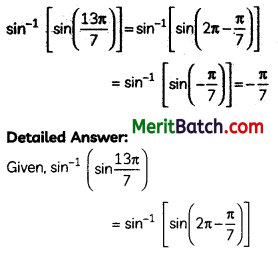OR
Let y ∈ N (codomain). Then ∃ 2y ∈ N (domain) such that f(2y) = 2yy = y.
Hence, f is surjective.
1, 2 ∈ N (domain) such that f(1) = 1 = f(2)
Hence, f is not injective.

Question 22.

A man 1.6 m tall walks at the rate of 0.3 m/s away from a street light that is 4 m above the ground. At what rate is the tip of his shadow moving? At what rate is his shadow lengthening? 

Solution:
Let AB represent the height of the street light from the ground. At any time t seconds.
let the man represented as ED of height 1.6 m be at a distance of x m from AB and the length of his shadow EC be y m.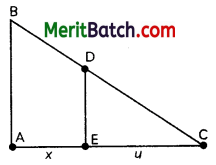Using the similarity of triangles, we have
41.6=x+yy
⇒ 3y = 2x

Differentiating both sides w.r.t t, we get
3dydt=2dxdt
dydt=23×0.3
⇒ dydt = 0.2

At any time t seconds, the tip of his shadow is at a distance of (x + y) m from AB.
The rate at which the tip of his shadow moves (dxdt+dydt) m/s = 0.5 m/s
The rate at which his shadow is lengthening = dydt m/s = 0.2 m/s

Question 23.

If a⃗ =i^−j^+7k^ and b⃗ =5i^−j^+λk^, then find the value of λ, so that vectors a⃗ +b⃗  and a⃗ −b⃗  are orthogonal. 

OR

Find the direction ratio and direction cosines of a line parallel to the line whose equations are 6x – 12 = 3y + 9 = 2z – 2.

Solution:

The equations of the line are 6x – 12 = 3y + 9 = 2z – 2, which when written in standard symmetric form, will be
x−216=y−(−3)13=z−112

Since lines are parallel, we have a1a2=b1b2=c1c2
Hence, the required direction ratios are (16,13,12) or (1, 2, 3)
and the required cosines are (114√,214√, and 314√)

Question 24.

If y1−x2−−−−−√+x1−y2−−−−−√ = 1, then prove that dydx=−1−y21−x2−−−−√ 

Solution:

y1−x2−−−−−√+x1−y2−−−−−√ = 1
Let sin-1 x = A and sin-1 y = B.
Then x = sin A and y = sin B
y1−x2−−−−−√+x1−y2−−−−−√ = 1

⇒ sin B cos A + sin A cos B = 1
⇒ sin(A + B) = 1
⇒ A + B = sin-1 1 = π2
⇒ sin-1 x + sin-1 y = π2

Differentiating w.r. to x, we obtain
dydx=−1−y21−x2−−−−√

### Section – C (18 marks)

(This section comprises short answer type questions (SA) of 3 marks each)

Question 26.

Find ∫dx3−2x−x2√ 

Solution: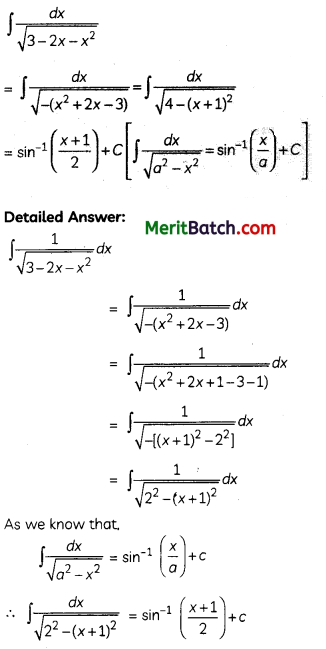Question 27.

Three friends go for coffee. They decide who will pay the bill, by each tossing a coin and then letting the “odd person” pay. There is no odd person if all three tosses produce the same result. If there is no odd person in the first round, they make the second round of tosses and continue to do so until there is an odd person. What is the probability that exactly three rounds of tosses are made? 

OR

Find the mean number of defective items in a sample of two items drawn one-by-one without replacement from an urn containing 6 items, which include 2 defective items. Assume that the items are identical in shape and size.

Solution:

P(not obtaining an odd person in a single round) = P(All three of them throw tails or All three of them throw heads)

= 12×12×12×2
= 14

P(obtaining an odd person in a single round) = 1 – P(not obtaining an odd person in a single round) = 34
The required probability = P(In the first round there is no odd person and ‘In the second round there is no odd person’ and ‘In the third round, there is an odd person)

= 14×14×34
= 364

OR

Let X denote the Random Variable defined by the number of defective items.

P(X=0) = 46×35=25
P(X=1) = 2×(26×45)=815
P(X=2) = 26×15=115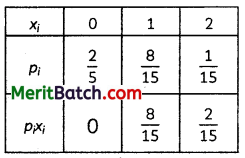Mean = Σpixi = 1015=23

Question 28.

Evaluate ∫π/3π/6dx1+tanx√ 
OR
Evaluate ∫40|x−1|dx

Solution:

Let I = ∫π/3π/6dx1+tanx√Question 29.

Solve the differential equation: y dx + (x – y2) dy = 0 

OR

Solve the differential equation: x dy – y dx = x2+y2−−−−−−√dx

Solution:

y dx + (x – y2) dy = 0

Reducing the given differential equation to the form dxdy + Px = Q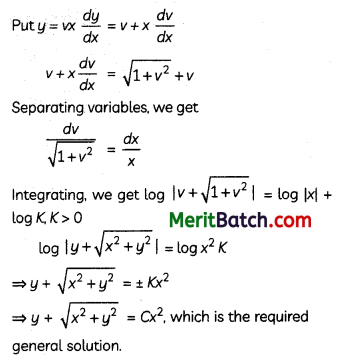Question 30.

Solve the following Linear Programming Problem graphically: 
Maximize Z = 400x + 300y subject to x + y ≤ 200, x ≤ 40, x ≥ 20, y ≥ 0

Solution:

We have Z = 400x + 300y subject to x + y ≤ 200, x ≤ 40, x ≥ 20, y ≥ 0
The corner points of the feasible region are C(20, 0), D(40, 0), B(40, 160), A(20, 180)Maximum profit occurs at x = 40, y = 160
and the maximum profit = ₹ 64,000

Question 31.

Find ∫(x3+x+1)(x2−1)dx 
Solution: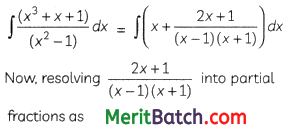### Section – D (20 marks)

(This Section comprises of Long answer type question (LA) of 5 marks)

Question 32.

Make a rough sketch of the region {(x, y): 0 ≤ y ≤ x2, 0 ≤ y ≤ x, 0 ≤ x ≤ 2} and find the area of the region using integration.

Solution:Question 33.

Define the relation R. in the set N × N as follows: 

For (a, b), (c, d) ∈ N × N, (a, b) R (c, d) if ad = bc. Prove that R is an equivalence relation in N × N.

OR

Given a non-empty set X, define the relation R in P(X) as follows:

For A, B ∈ P(X), (A, B) ∈ R if A ⊂ B. Prove that R s reflexive, transitive, and not symmetric.

Solution:

Let (a, b) ∈ N × N. Then we have
ab = ba (by commutative property of multiplication of natural numbers)
⇒ (a, b) R (a, b)
Hence, R is reflexive.
Let (a, b), (c, d) ∈ N × N such that (a, b) R (c, d).
⇒ cb = da (by commutative property of multiplication of natural numbers)
⇒ (c, d) R (a, b)
Hence, R is symmetric.
Let (a, b), (c, d), (e, f) ∈ N × N such that
(a, b) R (c, d) and (c, d) R (e, f).
Then ad = bc, cf = de
⇒ af = be
⇒ (a, b) R (e, f)
Hence, R is transitive.
Since, R is reflexive, symmetric, and transitive, R is an equivalence relation on N × N.

OR

Let A ∈ P(X). Then A ⊂ A
⇒ (A, A) ∈ R
Hence, R is reflexive.
Let A, B, C ∈ P(X) such that
(A, B), (B, C) ∈ R
⇒ A ⊂ B, B ⊂ C
⇒ A ⊂ C
⇒ (A, C) ∈ R
Hence, R is transitive.
Φ, X ∈ P(X) such that Φ ⊂ X.
Hence (Φ, X) ∈ R. But X ⊄ Φ
which implies that (X, Φ) ∉ R
Thus, R is not symmetric.

Question 34.

An insect is crawling along the line r⃗ =6i^+2j^+2k^+λ(i^−2j^+2k^) and another insect is crawling along the line r⃗ =−4i^−k+μ(3i^−2j^−2k^). At what points on the lines should they reach so that the distance between them is the shortest? Find the shortest possible distance between them. 

OR

The equations of motion of a rocket are x = 2t, y = -4t, z = 4t, where the time t is given in seconds and the coordinates of a moving point in km. what is the path of the rocket? At what distances will the rocket be from the starting point O(0, 0, 0) and from the following line in 10 seconds?

r⃗ =20i^−10j^+40k^+μ(10i^−20j^+10k^)

Solution:

The given lines are non-parallel lines. There is a unique line segment PQ (P lying on one and Q on the other, which is at right angles to both lines.

PQ is the shortest distance between the lines. Hence, the shortest possible distance between the insects = PQ
The position vector of P lying on the line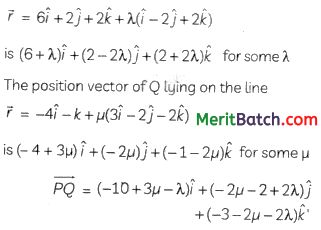Since PQ is perpendicular to both lines

(-10 + 3µ – λ) + (-2µ – 2 + 2λ)(-2) + (-3 – 2µ – 2λ) . 2 = 0
i.e, µ – 3λ = 4 …..(i)
and (-10 + 3µ – λ)3 + (-2µ – 2 + 2λ) (- 2) + (-3 – 2µ – 2λ) (-2) = 0
i.e., 17µ – 3λ = 20 ……..(ii)

solving (i) and (ii) for λ and µ, we get µ = 1, λ = -1.

The position vector of the points, at which they should be so that the distance between them is the shortest are 5i^+4j^ and −i^−2j^−3k^

PQ−→−=−6i^−6j^−3k^

The shortest distance = |PQ−→−|=62+62+32−−−−−−−−−−√=9

OR

Eliminating t between the equations, we obtain the equation of the path x2=y−4=z4, which are
the equations of the line passing through the origin having direction ratios < 2, -4, 4 >. This line is the path of the rocket.
When t = 10 seconds, the rocket will be at the point (20, -40, 40).

Hence, the required distance from the origin at 10 seconds = 202+402+402−−−−−−−−−−−−√ km
= 20 × 3 km
= 60 km
The distance of the point (20, -40, 40) from the given lineQuestion 35.

If A = ⎡⎣⎢231−3215−4−2⎤⎦⎥, find A-1. Use A-1 to solve the following system of equations
2x – 3y + 5z = 11, 3x + 2y – 4z = -5, x + y – 2z = -3. 
Solution:Section – E (12 marks)

This section comprises 3 Case-Study/Passage-based questions of 4 marks each with two Sub-Parts, First two Case-Study questions have three Sub-Parts (A), (B), (C) of marks 1, 1, 2 respectively. The third Case-Study question has two Sub-Parts of 2 marks each.

Question 36.

Case Study 1: Read the following passage and answer the questions given below. 
The temperature of a person during an intestinal illness is given by f(x) = -0.1×2 + mx + 98.6, 0 ≤ x ≤ 12, m is a constant, where f(x) is the temperature in °F at x days.

(A) Is the function differentiable in the interval (0, 12)? Justify your answer.
(B) If 6 is the critical point of the function, then find the value of the constant m.
(C) Find the intervals in which the function is strictly increasing/strictly decreasing.

OR

Find the points of Local maximum/local minimum, if any, in the interval (0, 12) as well as the points of absolute maximum/absolute minimum in the interval [0, 12]. Also, find the corresponding local maximum/local minimum and the absolute maximum/absolute minimum values of the function.

Solution:

(A) f(x) = -0.1x2 + mx + 98.6, is a polynomial function that is differentiable everywhere, hence, differentiable in (0, 12).
(B) f'(x) = -0.2x + m

Since 6 is the critical point.

(C) f(x) = -0.1x2 + 1.2x + 98.6
f(x) = -0.2x + 1.2 = -0.2(x – 6)OR

f(x) = -0.1x2 + 1.2x + 98.6,
f(x) = -0.2x + 1.2,
f(6) = 0,
f'(x) = -0.2
f'(6) = -0.2 < 0

Hence, by the second derivative test 6 is a point of the local maximum. The local maximum value = f(6)
= -0.1 × 62 + 1.2 × 6 + 98.6
= 102.2

We have f(0) = 98.6, f(6) = 102.2, f(12) = 98.6
6 is the point of absolute maximum and the absolute maximum value of the function = 102.2.
0 and 12 are both the points of absolute minimum and the absolute minimum value of the function = 98.6.

Question 37.

Case Study 2: Read the following passage and answer the questions given below.For an elliptical sports field, the authority wants to design a rectangular soccer field with the maximum possible area. The sports field is given by the graph of x2a2+y2b2=1.

(A) If the length and the breadth of the rectangular field be 2x and 2y respectively, then find the area function in terms of x.

(B) Find the critical point of the function.

(C) Use the first derivative test to find the length 2x and width 2y of the soccer field (in terms of a and b) that maximize its area.

OR

Use the second derivative test to find the length 2x and width 2y of the soccer field (in terms of a and b) that maximize its area.

Solution:(C) For the values of x less than a2√ and close to a2√,dAdx>0 and for the values of x greater than a2√ and close to a2√,dAdx<0.

Hence, by the first derivative test, there is a local maximum at the critical point x = a2√
Since there is only one critical point, therefore, the area of the soccer field is maximum at this critical point x = a2√
Thus, for the maximum area of the soccer field, its length should be a√2 and its width should be b√2.

OR

A = 2x × 2baa2−x2−−−−−−√, x ∈ (0, a)
Squaring both sides, we get
Z = A2 = 16b2a2x2(a2−x2)=16b2a2(x2a2−x4), x ∈ (0, a)
A is maximum when Z is maximum.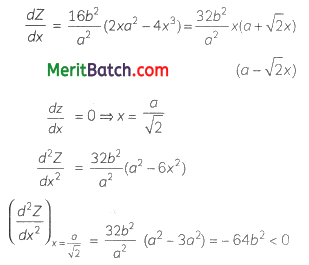Hence, by the second derivative test, there is a local maximum value of Z at the critical point x = a2√.
Since there is only one critical point, therefore, Z is maximum at x = a2√,
hence, A is maximum at x = a2√
Thus, for the maximum area of the soccer field, its length should be a√2 and its width should be b√2.

Question 38.

Case Study 2: Read the following passage and answer the questions given below.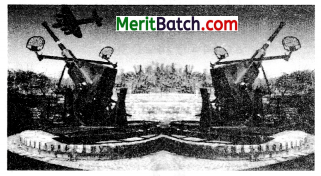There are two antiaircraft guns, named A and B. The probabilities that the shell fired from them hit an airplane are 0.3 and 0.2 respectively.

Both of them fired one shell at an airplane at the same time.

(A) What is the probability that the shell fired from exactly one of them hit the plane?
(B) If it is known that the shell fired from exactly one of them hit the plane, then what is the probability that it was fired from B?

Solution:

(A) Let P be the event that the shell fired from A hits the plane and Q be the event that the shell fired from B hits the plane.

The following four hypotheses are possible before the trial with the guns operating independently:

E1=PQ,E2=P⃗ Q⃗ ,E3=P⃗ Q,E4=PQ⃗
Let E = The shell fired from exactly one of them hits the plane.
P(E1) = 0.3 × 0.2 = 0.06
P(E2) = 0.7 × 0.8 = 0.56
P(E3) = 0.7 × 0.2 = 0.14
P(E4) = 0.3 × 0.8 = 0.24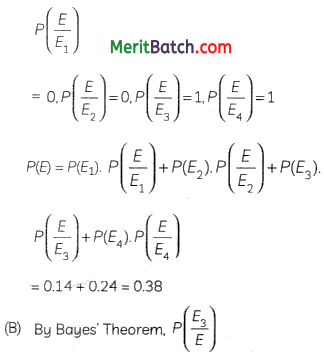Note: The four hypotheses form the partition of the sample space and it can be seen that the sum of their probabilities is 1. The hypotheses E1 and E2 are actually eliminated as P(EE1)=P(EE2)=0

An alternative way of writing the solution:

(A) P(Shell fired from exactly one of them hits the plane) = P[(Shell from A hits the plane and Shell from B does not hit the plane) or (Shell from A does not hit the plane and Shell from B hits the plane)]
= 0.3 × 0.8 + 0.7 × 0.2
= 0.38
(B) P(Shell fired from B hit the plane/Exactly one of them hit the plane)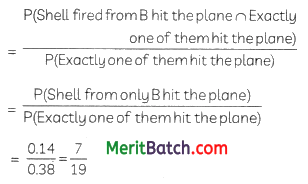## FAQ

Which Book Is Best For Class 12 Maths Board Exam?

NCERT Textbook

Is NCERT enough for Class 12 board Maths?

Yes

Is class 12 NCERT Maths difficult?

NCERT Maths of class 12 is not difficult. Practice makes you perfect#### Robin Singh

Robin Singh is education professional tutor of NCERT. I have good knowledge of CBSE all subjects. Expert in maths, physics and chemistry. if students have any doubt about NCERT Solutions so contact us info@cbsenotes.in.

View all posts by Robin Singh →